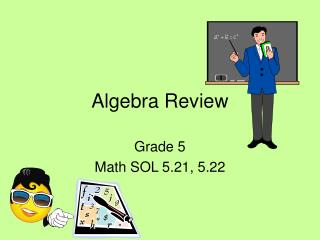DownloadDownload PresentationAlgebra Review

# Algebra Review

Télécharger la présentation## Algebra Review

- - - - - - - - - - - - - - - - - - - - - - - - - - - E N D - - - - - - - - - - - - - - - - - - - - - - - - - - -
##### Presentation Transcript

1. Algebra Review Grade 5 Math SOL 5.21, 5.22

2. 1. Which represents the unknown value in the following statement? A. f B. 6 18

3. 2. Jewel rode 3 more miles on her bike than Caleb. If m represents the number of miles Caleb rode, which could be used to determine the number of miles Jewel rode? A. B. C. D.

4. 3. A number machine uses a rule to change numbers. The table shows the results. Which could be the rule the number machine used? A. g + 7 = m B. g 7 = m C. g x 7 = m D. g - 7 = m

5. 4. Which of the following can be solved using the open sentence r – 4 = ? A. B. C. D. Kera has 4 times more notebooks than Bill. If r represents the number of notebooks Bill has, how many notebooks does Kera have? Bill has 4 times more notebooks than Kera. If r represents the number of notebooks Bill has, how many notebooks does Kera have? Kera has 4 fewer notebooks than Bill. If r represents the number of notebooks Bill has, how many notebooks does Kera have? Kera has 4 more notebooks than Bill. If r represents the number of notebooks Bill has, how many notebooks does Kera have?

6. 5. Chase earned \$3 for each car he washed at the fundraiser. At the end of the day, he had collected \$90.00. What does the z represent in the equation 3z = 90? A. The number of hours Chase worked at the fundraiser. B. The number of cars Chase washed at the fundraiser. C. The amount of money Chase made for each car he washed. D. The amount of money Chase made when the fundraiser ended.

7. 6. Kiara has 5 times the amount of Wii games than Darius owns. If Darius’ Wii games are represented by w, which expression best represents the amount of Wii games Kiara has? A. B. C. D.